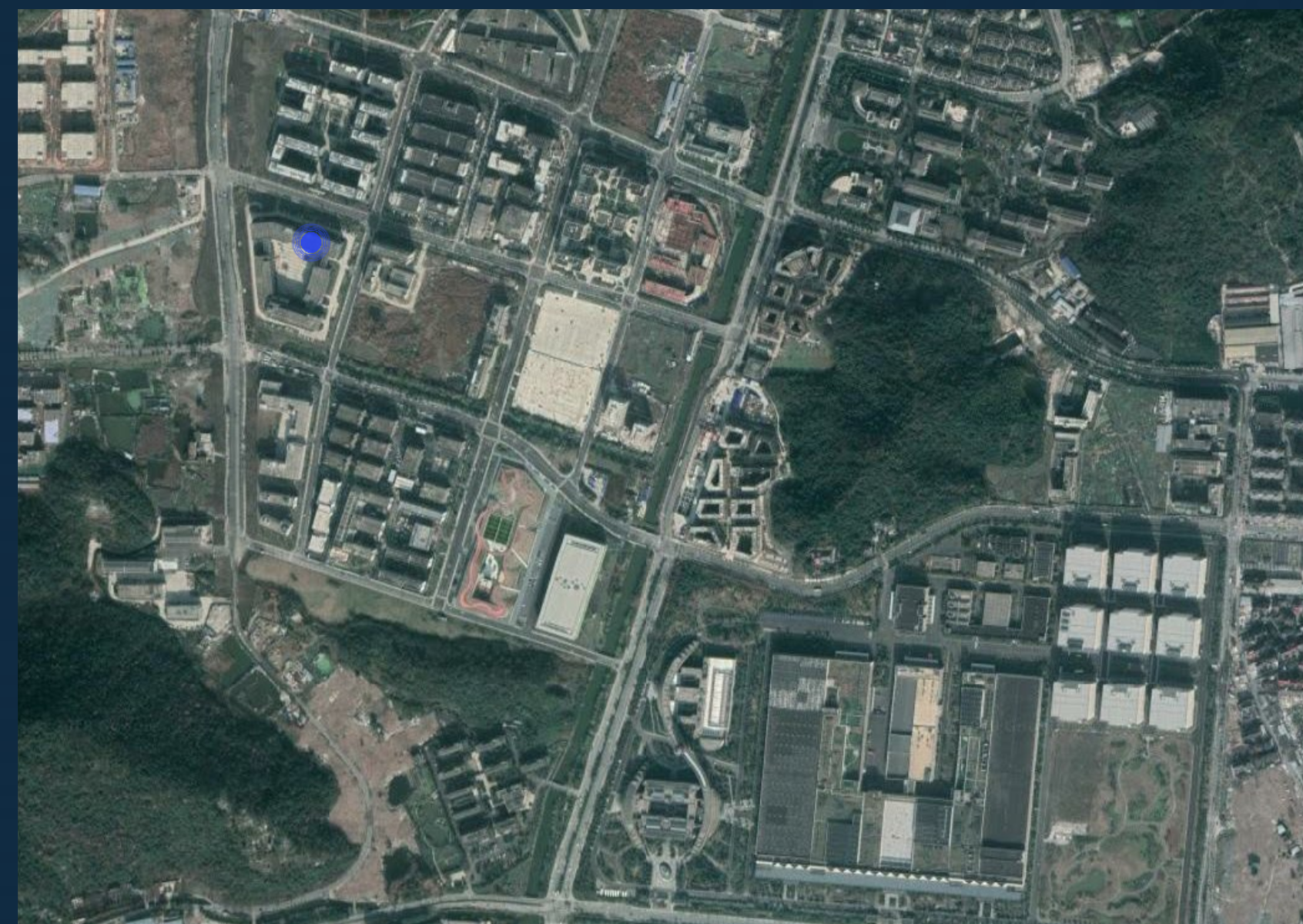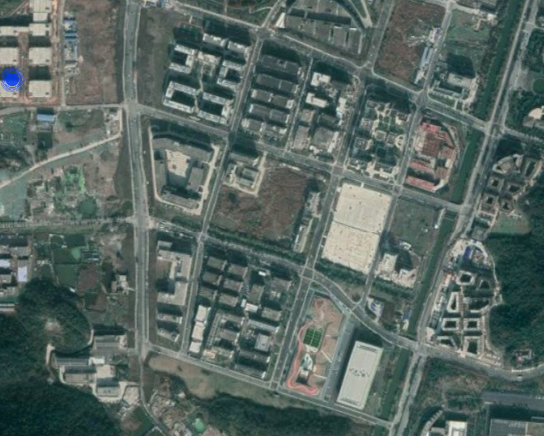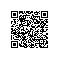# DataV动态GPS数据源在高德地图上坐标转换方案// 以下是简化的转换方法wgs84togcj02
var PI = 3.1415926535897932384626;
var a = 6378245.0;
var ee = 0.00669342162296594323;

function transform_latitude(lng, lat) {
var lat = +lat;
var lng = +lng;
var ret = -100.0 + 2.0 * lng + 3.0 * lat + 0.2 * lat * lat + 0.1 * lng * lat + 0.2 * Math.sqrt(Math.abs(lng));
ret += (20.0 * Math.sin(6.0 * lng * PI) + 20.0 * Math.sin(2.0 * lng * PI)) * 2.0 / 3.0;
ret += (20.0 * Math.sin(lat * PI) + 40.0 * Math.sin(lat / 3.0 * PI)) * 2.0 / 3.0;
ret += (160.0 * Math.sin(lat / 12.0 * PI) + 320 * Math.sin(lat * PI / 30.0)) * 2.0 / 3.0;
return ret
};

function transform_longitude(lng, lat) {
var lat = +lat;
var lng = +lng;
var ret = 300.0 + lng + 2.0 * lat + 0.1 * lng * lng + 0.1 * lng * lat + 0.1 * Math.sqrt(Math.abs(lng));
ret += (20.0 * Math.sin(6.0 * lng * PI) + 20.0 * Math.sin(2.0 * lng * PI)) * 2.0 / 3.0;
ret += (20.0 * Math.sin(lng * PI) + 40.0 * Math.sin(lng / 3.0 * PI)) * 2.0 / 3.0;
ret += (150.0 * Math.sin(lng / 12.0 * PI) + 300.0 * Math.sin(lng / 30.0 * PI)) * 2.0 / 3.0;
return ret
};

/**
* WGS84转GCj02
* @param lng
* @param lat
* @returns {*[]}
*/
function wgs84togcj02(lng, lat) {
var lat = +lat;
var lng = +lng;
var dlat = transform_latitude(lng - 105.0, lat - 35.0);
var dlng = transform_longitude(lng - 105.0, lat - 35.0);
var radlat = lat / 180.0 * PI;
magic = 1 - ee * magic * magic;
var sqrtmagic = Math.sqrt(magic);
dlat = (dlat * 180.0) / ((a * (1 - ee)) / (magic * sqrtmagic) * PI);
dlng = (dlng * 180.0) / (a / sqrtmagic * Math.cos(radlat) * PI);
var mglat = lat + dlat;
var mglng = lng + dlng;
return [mglng, mglat]
};

// 以下是过滤脚本，调用wgs84togcj02转换方法
var output = [];

for (var item in data) {
var d = { "time": "", "longitude": "", "latitude": "", "data": "1", "type": "1" };

var longitude_degree = parseInt(LocatorPayLoadData.substring(6, 10), 16);
var longitude_minute = parseInt(LocatorPayLoadData.substring(10, 12), 16);
var longitude_second = parseInt(LocatorPayLoadData.substring(12, 16), 16);
var latitude_degree = parseInt(LocatorPayLoadData.substring(16, 18), 16);
var latitude_minute = parseInt(LocatorPayLoadData.substring(18, 20), 16);
var latitude_second = parseInt(LocatorPayLoadData.substring(20, 24), 16);
var date = new Date(parseInt(data[item].time));
var year = date.getFullYear(),
month = date.getMonth() + 1,//月份是从0开始的
day = date.getDate(),
hour = date.getHours(),
min = date.getMinutes(),
sec = date.getSeconds();
var newTime = year + '-' +
(month < 10 ? '0' + month : month) + '-' +
(day < 10 ? '0' + day : day) + ' ' +
(hour < 10 ? '0' + hour : hour) + ':' +
(min < 10 ? '0' + min : min) + ':' +
(sec < 10 ? '0' + sec : sec);
d.time = newTime;
d.longitude = longitude_degree + longitude_minute / 60 + longitude_second / (3600 * 100);
d.latitude = latitude_degree + latitude_minute / 60 + latitude_second / (3600 * 100);
var longitude_wgs84 = d.longitude.toFixed(6);
var latitude_wgs84 = d.latitude.toFixed(6);
var result = wgs84togcj02(longitude_wgs84, latitude_wgs84);
d.longitude = result.toFixed(6)
d.latitude = result.toFixed(6)

output.push(d);
}

return output;

## 术语

### WSG84

WGS的最新版本为WGS 84（也称作WGS 1984、EPSG:4326），1984年定义、最后修订于2004年。之前的版本有WGS 72、WGS 66、WGS 60。全球定位系统使用的就是WGS 84参考系。

## 鸣谢IoT使用钉钉扫一扫加入圈子
+ 订阅